Courses
Courses for Kids
Free study material
Offline Centres
More

# NCERT Solutions for Class 7 Maths Chapter 9 - In HindiLast updated date: 29th Nov 2023
Total views: 426.9k
Views today: 5.26k

## NCERT Solutions for Class 7 Maths Chapter 9 Rational Numbers In Hindi PDF Download

Download the Class 7 Maths NCERT Solutions in Hindi medium and English medium as well offered by the leading e-learning platform Vedantu. If you are a student of Class 7, you have reached the right platform. The NCERT Solutions for Class 7 Maths in Hindi provided by us are designed in a simple, straightforward language, which are easy to memorise. You will also be able to download the PDF file for NCERT Solutions for Class 7 Maths in Hindi from our website at absolutely free of cost.

NCERT, which stands for The National Council of Educational Research and Training, is responsible for designing and publishing textbooks for all the classes and subjects. NCERT textbooks covered all the topics and are applicable to the Central Board of Secondary Education (CBSE) and various state boards.

We, at Vedantu, offer free NCERT Solutions in English medium and Hindi medium for all the classes as well. Created by subject matter experts, these NCERT Solutions in Hindi are very helpful to the students of all classes.

## प्रश्नावली 9.1

1: निम्नलिखित परिमेय संख्याओं के बीच में पांच परिमेय संख्याएं लिखिए:

(i) $-\text{1}$ और $0$

उत्तर: $-\text{1}$ और $0$

आइए हम भाजक $\text{6}$  के साथ तर्क संगत संख्याओं के रूप में $-\text{1}$ और $0$ लिखे।

=>${\text{-1}}$ = $\dfrac{-6}{6}$ और ${\text{-1}}$ = $\dfrac{0}{6}$

तो, $\dfrac{-6}{6}$ < $\dfrac{-5}{6}$ < $\dfrac{-4}{6}$ < $\dfrac{-3}{6}$ < $\dfrac{-2}{6}$ < $\dfrac{-1}{6}$ < $\dfrac{0}{6}$

=> ${\text{-1}}$ < $\dfrac{-5}{6}$ < $\dfrac{-4}{6}$ < $\dfrac{-3}{6}$ < $\dfrac{-2}{6}$ < $\dfrac{-1}{6}$ < ${\text{0}}$

इसलिए, $-\text{1}$ और $0$ के बीच पांच तर्क संगत संख्याएं हैं:

$\dfrac{-5}{6}$ < $\dfrac{-4}{6}$ < $\dfrac{-3}{6}$ < $\dfrac{-2}{6}$ < $\dfrac{-1}{6}$

(ii) $-\text{2}$ और $-\text{1}$

उत्तर: $-\text{2}$ और $-\text{1}$

आइए हम भाजक $\text{6}$ के साथ तर्क संगत संख्याओं के रूप में $-\text{2}$ और $-\text{1}$ लिखे ।

=> $-\text{2}$ = $\dfrac{-12}{6}$ और $-\text{1}$ = $\dfrac{-6}{6}$

तो, $\dfrac{-12}{6}$ < $\dfrac{-11}{6}$ < $\dfrac{-10}{6}$ < $\dfrac{-9}{6}$ < $\dfrac{-8}{6}$ < $\dfrac{-7}{6}$ < $\dfrac{-6}{6}$

=> ${\text{-2}}$ < $\dfrac{-11}{6}$ < $\dfrac{-5}{3}$ < $\dfrac{-3}{2}$ < $\dfrac{-4}{3}$ < $\dfrac{-7}{6}$ < ${\text{-1}}$

इसिलिये, $-\text{2}$ और $-\text{1}$ के बीच पांच तर्क संगत संख्याएं हैं:

${\text{-2}}$ < $\dfrac{-11}{6}$ < $\dfrac{-5}{3}$ < $\dfrac{-3}{2}$ < $\dfrac{-4}{3}$ < $\dfrac{-7}{6}$ < ${\text{-1}}$

(iii) $\dfrac{-4}{5}$और $\dfrac{-2}{3}$

उत्तर: $\dfrac{-4}{5}$ और $\dfrac{-2}{3}$

आइए हम एक ही भाजक के साथ तर्क संगत संख्याओं के रूप में  $\dfrac{-4}{5}$ और $\dfrac{-2}{3}$ लिखे।

=> $\dfrac{-4}{5}$ = $\dfrac{-36}{45}$ और $\dfrac{-2}{3}$ = $\dfrac{-30}{45}$

=> $\dfrac{-36}{45}$ < $\dfrac{-35}{45}$ < $\dfrac{-34}{45}$ < $\dfrac{-33}{45}$ < $\dfrac{-32}{45}$ < $\dfrac{-31}{45}$ < $\dfrac{-30}{45}$

=> $\dfrac{-4}{5}$ < $\dfrac{-7}{9}$ < $\dfrac{-34}{45}$ < $\dfrac{-11}{15}$ < $\dfrac{-32}{45}$ <  $\dfrac{-31}{45}$ < $\dfrac{-2}{3}$

Hence, the five rational numbers between $\dfrac{-4}{5}$ and $\dfrac{-2}{3}$ are:

$\dfrac{-4}{5}$ < $\dfrac{-7}{9}$ < $\dfrac{-34}{45}$ < $\dfrac{-11}{15}$ < $\dfrac{-32}{45}$ <  $\dfrac{-31}{45}$ < $\dfrac{-2}{3}$

(iv) $\dfrac{-1}{2}$ और $\dfrac{2}{3}$

उत्तर: $\dfrac{-1}{2}$ और $\dfrac{2}{3}$

आइए हम एक ही भाजक के साथ तर्क संगत संख्याओं के रूप में $\dfrac{-1}{2}$ और $\dfrac{2}{3}$

=> $\dfrac{-1}{2}$ = $\dfrac{-3}{6}$ और $\dfrac{2}{3}$ = $\dfrac{4}{5}$

=> $\dfrac{-3}{6}$ < $\dfrac{-2}{6}$ < $\dfrac{-1}{6}$ < $\dfrac{0}{6}$ < $\dfrac{1}{6}$ < $\dfrac{2}{6}$ < $\dfrac{3}{6}$ < $\dfrac{4}{6}$

=> $\dfrac{-1}{2}$ < $\dfrac{-1}{3}$ < $\dfrac{-1}{6}$ < ${\text{0}}$ < $\dfrac{1}{6}$ < $\dfrac{1}{3}$ < $\dfrac{1}{2}$ < $\dfrac{2}{3}$

इसलिए, $\dfrac{-1}{2}$ और $\dfrac{2}{3}$ के बीच पांच तर्क संगत संख्याएं हैं:

$\dfrac{-1}{3}$ < $\dfrac{-1}{6}$ < ${\text{0}}$ < $\dfrac{1}{6}$ < $\dfrac{1}{3}$

2: निम्नलिखित प्रतिरूपों मेंसेप्रत्येक चार और परिमेय सँख्याएलिखिए:

(i)$\dfrac{-3}{5}, \dfrac{-6}{10}, \dfrac{-9}{12}, \dfrac{-12}{20}$

उत्तर: (i) $\dfrac{-3}{5}, \dfrac{-6}{10}, \dfrac{-9}{12}, \dfrac{-12}{20} \ldots \ldots \ldots .$

= $\dfrac{\text{-3 * 1}}{\text{5 * 1}}, \dfrac{\text{-3 * 2}}{\text{5 * 2}}, \dfrac{\text{-3 * 3}}{\text{5 * 3}}, \dfrac{\text{-3 * 4}}{\text{5 * 4}},\ldots \ldots \ldots \ldots$

इसलिए, इस पैटर्न के अगले चार तर्क संगत संख्या हैं:

$\dfrac{\text{-3 * 5}}{\text{5 * 5}}, \dfrac{\text{-3 * 6}}{\text{5 * 6}}, \dfrac{\text{-3 * 7}}{\text{5 * 7}}, \dfrac{\text{-3 * 8}}{\text{5 * 8}}$

=$\dfrac{-15}{25}, \dfrac{-18}{30}, \dfrac{-21}{35}, \dfrac{-24}{40}$

(ii) $\dfrac{-1}{54}, \dfrac{-2}{8}, \dfrac{-3}{12}$

उत्तर: $\dfrac{-1}{54}, \dfrac{-2}{8}, \dfrac{-3}{12} \ldots \ldots \ldots \ldots \ldots$

= $\dfrac{\text{-1 * 1}}{\text{4 * 1}}, \dfrac{\text{-1 * 2}}{\text{4 * 2}}, \dfrac{\text{-1 * 3}}{\text{4 * 3}}......$

इसलिए, इस पैटर्न अगले चार तर्क संगत संख्या हैं:

$\dfrac{\text{-1 * 4}}{\text{4 * 4}}, \dfrac{\text{-1 * 5}}{\text{4 * 5}}, \dfrac{\text{-1 * 6}}{\text{4 * 6}}, \dfrac{\text{-1 * 7}}{\text{4 * 7}}$

=$\dfrac{-4}{16}, \dfrac{-5}{20}, \dfrac{-6}{24}, \dfrac{-7}{28}$

(iii)$\dfrac{-1}{6}, \dfrac{2}{-12}, \dfrac{3}{-18}, \dfrac{4}{-24}$

उत्तर: $\dfrac{-1}{6}, \dfrac{2}{-12}, \dfrac{3}{-18}, \dfrac{4}{-24} \ldots \ldots \ldots .$

= $\dfrac{\text{-1 * 1}}{\text{6 * 1}}, \dfrac{\text{1 * 2}}{\text{-6 * 2}}, \dfrac{\text{1 * 3}}{\text{-6 * 3}}, \dfrac{\text{1 * 4}}{\text{-6 * 4}},\ldots \ldots \ldots \ldots$

इसलिए, इसके अगले चार तर्क संगत संख्या हैं:

$\dfrac{\text{1 * 5}}{\text{-6 * 5}}, \dfrac{\text{1 * 6}}{\text{-6 * 6}}, \dfrac{\text{1 * 7}}{\text{-6 * 7}}, \dfrac{\text{1 * 8}}{\text{-6 * 8}}$

=$\dfrac{5}{-30}, \dfrac{6}{-36}, \dfrac{7}{-42}, \dfrac{8}{-42}$

(iv) $\dfrac{-2}{3}, \dfrac{2}{-3}, \dfrac{4}{-6}, \dfrac{6}{-9}$

उत्तर: $\dfrac{-2}{3}, \dfrac{2}{-3}, \dfrac{4}{-6}, \dfrac{6}{-9} \ldots \ldots \ldots .$

=> $\dfrac{\text{-2 * 1}}{\text{3 * 1}}, \dfrac{\text{2 * 1}}{\text{-3 * 1}}, \dfrac{\text{2 * 1}}{\text{-3 * 1}}, \dfrac{\text{2 * 1}}{\text{-3 * 1}},\ldots \ldots \ldots \ldots$

इसलिए, इस पैटर्न के अगले चार तर्क संगत संख्या हैं:

$\dfrac{\text{2 * 4}}{\text{-3 * 4}}, \dfrac{\text{2 * 5}}{\text{-3 * 5}}, \dfrac{\text{2 * 6}}{\text{-3 * 6}}, \dfrac{\text{2 * 7}}{\text{-3 * 7}}$

=$\dfrac{8}{-12}, \dfrac{10}{-15}, \dfrac{12}{-18}, \dfrac{14}{-21}$

3: निम्नलिखित के समतुल्य चार परिमेय सँख्याए लिखिए:

(i) $\dfrac{-2}{7}$

उत्तर: $\dfrac{-2}{7}$

$\dfrac{\text{(-2 * 2)}}{\text{(7 * 2)}} = \dfrac{-4}{14}, \dfrac{\text{(-2 * 3)}}{\text{(7 * 3)}} = \dfrac{-6}{21}, \dfrac{\text{(-2 * 4)}}{\text{(7 * 4)}} = \dfrac{-8}{28}, \dfrac{\text{(-2 * 5)}}{\text{(7 * 5)}} = \dfrac{-10}{35}$

इसलिए, चार तर्क संगत संख्याएँ हैं :

$\dfrac{-4}{14}$, $\dfrac{-6}{21}$, $\dfrac{-8}{28}$,  $\dfrac{-10}{35}$

(ii) $\dfrac{5}{-3}$

उत्तर: $\dfrac{5}{-3}$

$\dfrac{\text{(5 * 2)}}{\text{(-3 * 2)}} = \dfrac{10}{-6}, \dfrac{\text{(5 * 3)}}{\text{(-3 * 3)}} = \dfrac{15}{-9}, \dfrac{\text{(5 * 4)}}{\text{(-3 * 4)}} = \dfrac{20}{-12}, \dfrac{\text{(5 * 5)}}{\text{(-3 * 5)}} = \dfrac{25}{-15}$

इसलिए, चार तर्क संगत संख्याएँ हैं: -

$\dfrac{10}{-6}$, $\dfrac{15}{-9}$, $\dfrac{20}{-12}$,  $\dfrac{25}{-15}$

(iii)$\dfrac{4}{9}$

उत्तर: $\dfrac{4}{9}$

$\dfrac{\text{(4 * 2)}}{\text{(9 * 2)}} = \dfrac{8}{18}, \dfrac{\text{(4 * 3)}}{\text{(9 * 3)}} = \dfrac{12}{27}, \dfrac{\text{(4 * 4)}}{\text{(9 * 4)}} = \dfrac{16}{36}, \dfrac{\text{(4 * 5)}}{\text{(9 * 5)}} = \dfrac{20}{45}$

इसलिए, चार तर्क संगत संख्याएँ हैं: -

$\dfrac{8}{18}$, $\dfrac{12}{27}$, $\dfrac{16}{36}$,  $\dfrac{20}{45}$

4: एक संख्या रेखा खींचिए और उस पर निम्नलिखित परिमेयसंख्याओं कोनिरूपित कीजिये:

(i) $\dfrac{3}{4}$

उत्तर: $\dfrac{3}{4}$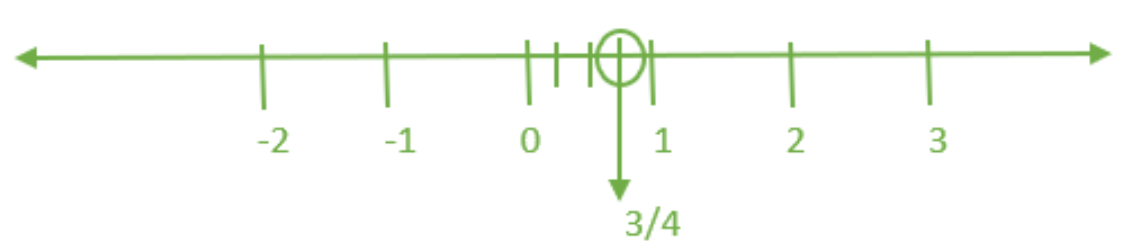(ii)$\dfrac{-5}{8}$

उत्तर: $\dfrac{-5}{8}$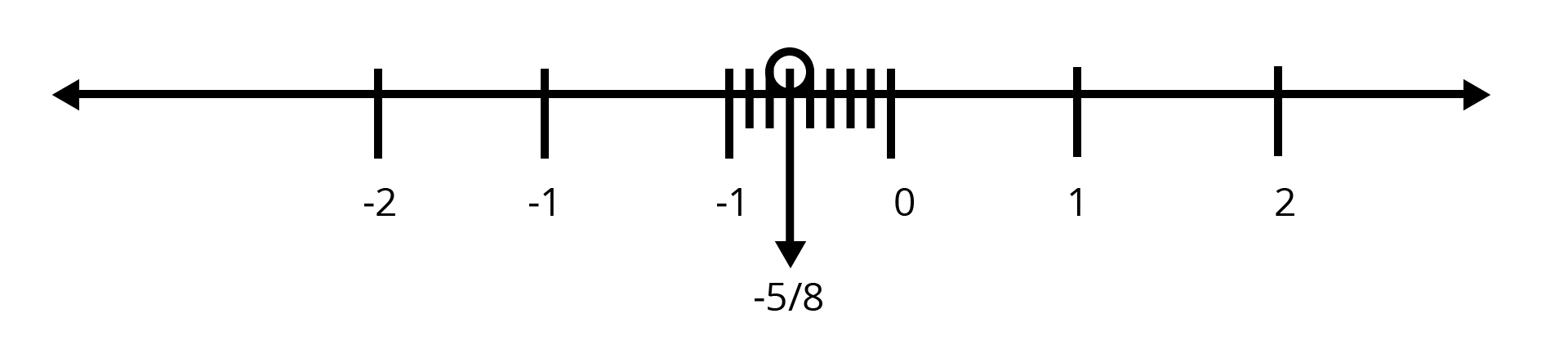(iii) $\dfrac{-7}{4}$

उत्तर: $\dfrac{-7}{4}$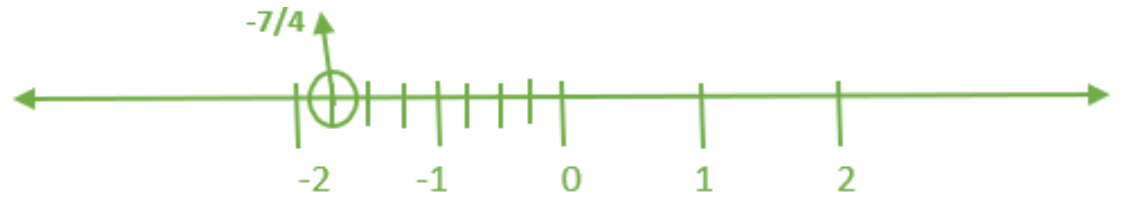(iv)$\dfrac{7}{8}$

उत्तर: $\dfrac{7}{8}$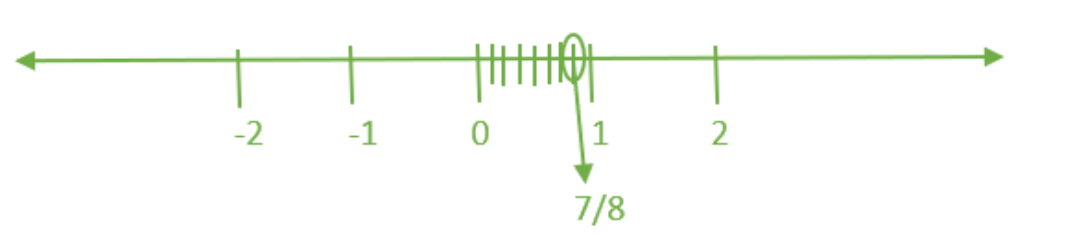5: एक संख्या रेखा पर बिंदु P, Q, S, T, U, A और B इस प्रकार हैं की TR=RS =SU तथा AP = PQ = QB है। P, Q, R और S सेनिरूपितपरिमेय संख्याओंको लिखिए।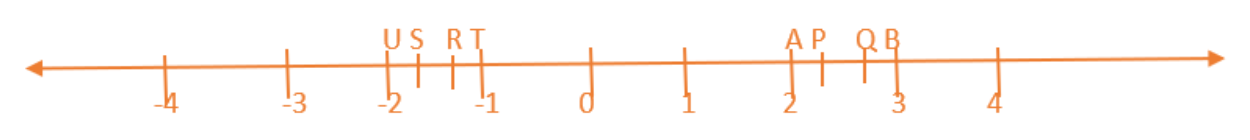उत्तर: प्रत्येक भाग जो दो संख्याओं के बीच है, को 3 भाग में विभाजित किया गया है।

अतः A  = $\dfrac{6}{3}$ , P = $\dfrac{7}{3}$ , Q = $\dfrac{8}{3}$ तथा B = $\dfrac{9}{3}$

इसी तरह, T  = $\dfrac{-3}{3}$ , R = $\dfrac{-4}{3}$ , S  = $\dfrac{-5}{3}$ तथा U = $\dfrac{-6}{3}$

इस प्रकार, तर्क संगत संख्याओं का प्रतिनिधित्व क्रमशः P, Q, R और S $\dfrac{7}{3}$, $\dfrac{8}{3}$, $\dfrac{-4}{3}$ और $\dfrac{-5}{3}$ है।

6: निम्नलिखितमें रो कौन रो जुगम एक ही परिमेय संख्या कोनिरूपित करतेहै ?

(i) $\dfrac{-7}{21}$ और $\dfrac{3}{9}$

उत्तर: $\dfrac{-7}{21}$ और $\dfrac{3}{9}$

$\dfrac{-7}{21}$ = $\dfrac{-1}{3}$ [ 7 और $\text{21}, \text{ 7}$ से विभाजितहैं]

$\dfrac{3}{9}$ = $\dfrac{1}{3}$ [ 3 और $\text{9}, \text{ 3}$ से विभाजितहैं]

क्योंकि, $\dfrac{-1}{3}$  $\ne$  $\dfrac{1}{3}$

So, $\dfrac{-7}{21}$  $\ne$  $\dfrac{3}{9}$

(ii) $\dfrac{-16}{20}$ और $\dfrac{20}{-25}$

उत्तर: $\dfrac{-16}{20}$ और $\dfrac{20}{-25}$

$\dfrac{-16}{20}$ = $\dfrac{-4}{5}$ [ 16 और $\text{2}0, \text{ 4}$ से विभाजितहैं]

$\dfrac{20}{-25}$ = $\dfrac{-20}{25}$ = $\dfrac{-4}{5}$  [ 20 और $\text{25}, \text{ 5}$ से विभाजितहैं ]

क्योंकि, $\dfrac{-4}{5}$  = $\dfrac{-4}{5}$

So, $\dfrac{-16}{20}$ = $\dfrac{20}{-25}$

(iii) $\dfrac{-2}{-3}$ और $\dfrac{2}{3}$

उत्तर: $\dfrac{-2}{-3}$ और $\dfrac{2}{3}$

$\dfrac{-2}{-3}$ = $\dfrac{2}{3}$

$\dfrac{2}{3}$ = $\dfrac{2}{3}$

क्योंकि, $\dfrac{2}{3}$ = $\dfrac{2}{3}$

So, $\dfrac{-2}{-3}$ = $\dfrac{2}{3}$

(iv) $\dfrac{-3}{5}$ और $\dfrac{-12}{20}$

उत्तर: $\dfrac{-3}{5}$ और $\dfrac{-12}{20}$

$\dfrac{-3}{5}$ = $\dfrac{-3}{5}$

$\dfrac{-12}{20}$ = $\dfrac{-3}{5}$ [ 12 और $\text{2}0, \text{ 4}$ से विभाजित हैं]

क्योंकि, $\dfrac{-3}{5}$ = $\dfrac{-3}{5}$

इसलिए, $\dfrac{-3}{5}$ = $\dfrac{-12}{20}$

(v) $\dfrac{8}{-5}$ और $\dfrac{-24}{15}$

उत्तर: $\dfrac{8}{-5}$ और $\dfrac{-24}{15}$

$\dfrac{8}{-5}$ = $\dfrac{-8}{5}$

$\dfrac{-24}{-5}$ = $\dfrac{-8}{5}$  [ 24 and 15 are divided by 3]

क्योंकि, $\dfrac{-8}{5}$ = $\dfrac{-8}{5}$

इसलिए, $\dfrac{8}{-5}$ = $\dfrac{-24}{15}$

(vi) $\dfrac{1}{3}$ और $\dfrac{-1}{9}$

उत्तर: $\dfrac{1}{3}$ और  $\dfrac{-1}{9}$

$\dfrac{1}{3}$ = $\dfrac{1}{3}$

$\dfrac{-1}{9}$ = $\dfrac{-1}{9}$

इसलिए,  $\dfrac{-5}{-9}$ $\ne$  $\dfrac{5}{-9}$

(vii) $\dfrac{-5}{9}$ और $\dfrac{5}{-9}$

उत्तर:  $\dfrac{1}{3}$ और  $\dfrac{-1}{9}$

$\dfrac{-5}{-9}$ = $\dfrac{5}{9}$

$\dfrac{5}{-9}$ = $\dfrac{-5}{9}$

क्योंकि, $\dfrac{5}{9}$  $\ne$ $\dfrac{-5}{9}$

इसलिए,  $\dfrac{-5}{-9}$ $\ne$  $\dfrac{5}{-9}$

7: निम्नलिखित परिमेय संख्याओं को उनके सरलतम रूप में लिखिए:

(i) $\dfrac{-8}{6}$

उत्तर: $\dfrac{-8}{6}$ = $\dfrac{-4}{3}$ [ 8 और $\text{6}, \text{ 2}$ से विभाजित हैं]

(ii) $\dfrac{25}{45}$

उत्तर: $\dfrac{25}{45}$ = $\dfrac{5}{9}$ [ 25 और $\text{45}, \text{ 5}$ से विभाजित हैं]

(iii) $\dfrac{-44}{72}$

उत्तर: $\dfrac{-44}{72}$ = $\dfrac{-11}{18}$ [ 44 और $\text{72}, \text{ 4}$ से विभाजित हैं]

(iv) $\dfrac{-8}{10}$

उत्तर: $\dfrac{-8}{10}$ = $\dfrac{-4}{5}$ [ 8 और $\text{10}, \text{ 2}$ से विभाजित हैं]

8: संकेतो > ,  < , और = मेंसेसही संकेत चुन कर रिक्त स्थानो कोभरिए:

(i) $\dfrac{-5}{7}$  $[ \,\ ]$  $\dfrac{2}{3}$

उत्तर: $\dfrac{-5}{7}$ [  <  ] $\dfrac{2}{3}$ क्योंकि धनात्मक संख्या ऋणात्मकसंख्या से अधिक है.

(ii) $\dfrac{-4}{5}$  $[ \,\ ]$  $\dfrac{-5}{8}$

उत्तर: $\dfrac{\left( -\text{4 }*\text{ 7} \right)}{\left( \text{5 }*\text{ 7} \right)}=\dfrac{-\text{ 28}}{\text{35}}$

$\dfrac{\left( -\text{5 }*\text{ 5} \right)}{\left( \text{7 }*\text{ 5} \right)}=\dfrac{-\text{ 25}}{\text{35}}$

क्योंकि, $\dfrac{-4}{5}$ [ <  ] $\dfrac{-5}{7}$

(iii) $\dfrac{-7}{8}$  $[ \,\ ]$  $\dfrac{14}{-16}$

उत्तर: $\dfrac{\left( -\text{7 }*\text{ 2} \right)}{\left( \text{8 }*\text{ 2} \right)}=\dfrac{-\text{ 14}}{\text{16}}$

क्योंकि, $\dfrac{-7}{8}$ [  =  ] $\dfrac{14}{-16}$

(iv) $\dfrac{-8}{5}$  $[ \,\ ]$  $\dfrac{-7}{4}$

उत्तर: $\dfrac{\left( -\text{8 }*\text{ 4} \right)}{\left( \text{5 }*\text{ 4} \right)}=\dfrac{-\text{ 32}}{\text{20}}$

$\dfrac{\left( -\text{7 }*\text{ 5} \right)}{\left( \text{4 }*\text{ 5} \right)}=\dfrac{-\text{ 35}}{\text{20 }}$

क्योंकि, $\dfrac{-8}{5}$ [  >  ] $\dfrac{-7}{4}$

(v) $\dfrac{1}{-3}$  $[ \,\ ]$  $\dfrac{-1}{4}$

उत्तर: $\dfrac{\left( \text{1 }*\text{ 4} \right)}{\left( -\text{3 }*\text{ 4} \right)}=\dfrac{\text{ 4}}{-\text{12 }}=\dfrac{-\text{4}}{\text{12}}$

$\dfrac{\left( -\text{1 }*\text{ 3} \right)}{\left( -\text{4 }*\text{ 3} \right)}=\dfrac{-\text{ 3}}{\text{13 }}$

क्योंकि, $\dfrac{1}{-3}$ [  <  ] $\dfrac{-1}{4}$

(vi) $\dfrac{5}{-11}$  $[ \,\ ]$  $\dfrac{-5}{11}$

उत्तर: $\dfrac{5}{-11}$ [ = ] $\dfrac{-5}{11}$

इसलिए,  $\dfrac{5}{-11}$ [ = ] $\dfrac{-5}{11}$

(vii) $0$  $[ \,\ ]$  $\dfrac{-7}{6}$

उत्तर: 0 [  >  ] $\dfrac{-7}{6}$ के बाद से $0$ नकारात्मक संख्या से अधिक है.

9: निम्नलखित में प्रत्येक में से कौन सी संख्या बड़ी है।

(i) $\dfrac{2}{3}, \dfrac{5}{2}$

उत्तर:

$\dfrac{2}{3} = \dfrac{2 \times 2}{3 \times 2}$

$\dfrac{5}{2} = \dfrac{5 \times 3}{2 \times 3}$

क्योंकि, $\dfrac{4}{6 } < \dfrac{15}{6}$

इसलिए, $\dfrac{2}{3} < \dfrac{5}{2}$

(ii) $\dfrac{-5}{6}, \dfrac{-4}{3}$

उत्तर: $\dfrac{-5}{6} = \dfrac{-5 \times 1}{6 \times 1}$

$\dfrac{-4}{3} = \dfrac{-4 \times 2}{3 \times 2}$

क्योंकि, $\dfrac{-5}{6 } > \dfrac{-8}{6}$

इसलिए, $\dfrac{-5}{6} > \dfrac{-4}{3}$

(iii) $\dfrac{-3}{4}, \dfrac{2}{-3}$

उत्तर: $\dfrac{-3}{4} = \dfrac{-3 \times 3}{4 \times 3}$

$\dfrac{-2}{3} = \dfrac{-2 \times 4}{3 \times 4}$

क्योंकि, $\dfrac{-9}{12 } < \dfrac{-8}{12}$

इसलिए, $\dfrac{-3}{4} < \dfrac{2}{-3}$

(iv) $\dfrac{-1}{4}, \dfrac{1}{4}$

उत्तर: $\dfrac{-1}{4 } < \dfrac{1}{4}$ के बाद से एक सकारात्मक संख्या एक नकारत्मकसंख्या से अधिक है.

(v) $\dfrac{-23}{7}, \dfrac{-19}{5}$

उत्तर: $\dfrac{-23}{7} = \dfrac{-23 \times 5}{7 \times 5}$

$\dfrac{-19}{5} = \dfrac{-19 \times 7}{5 \times 7}$

क्योंकि, $\dfrac{-115}{35 } > \dfrac{-133}{6}$

इसलिए, $\dfrac{-23}{7} > \dfrac{-19}{5}$

10: निम्नलखित परिमेय संख्याओं को आरोही क्रम में लिखिए।

(i) $\dfrac{-3}{5}, \dfrac{-2}{5}, \dfrac{-1}{5}$

उत्तर:  $\dfrac{-3}{5}, \dfrac{-2}{5}, \dfrac{-1}{5}$

$\dfrac{-3}{5} < \dfrac{-2}{5} < \dfrac{-1}{5}$

(ii) $\dfrac{1}{3}, \dfrac{-2}{9}, \dfrac{-4}{3}$

उत्तर: $\dfrac{1}{3}, \dfrac{-2}{9}, \dfrac{-4}{3}$

$\dfrac{3}{9}, \dfrac{-2}{9}, \dfrac{-12}{9}$ एक ही हर में कन्वर्ट करना

$\dfrac{-12}{9} < \dfrac{-2}{9} < \dfrac{3}{9}$

(iii) $\dfrac{-3}{7}, \dfrac{-3}{2}, \dfrac{-3}{4}$

उत्तर: $\dfrac{-3}{7}, \dfrac{-3}{2}, \dfrac{-3}{4}$

$\dfrac{-3}{2 } < \dfrac{-3}{4 } < \dfrac{-3}{7}$

## प्रश्नावली 9.2

1: योग ज्ञात कीजिए:

(i)$\dfrac{5}{4}+\left( \dfrac{-11}{4} \right)$

उत्तर: $\dfrac{5}{4}+(\dfrac{-11}{4})=\dfrac{5-11}{4}=\dfrac{-3}{2}$

(ii)$\dfrac{5}{3}+\dfrac{3}{5}$

उत्तर:

$\dfrac{5}{3}+\dfrac{3}{5}=\dfrac{5\text{x5}}{\text{3x5}}+\dfrac{\text{3x3}}{\text{5x3}}=\dfrac{34}{15}$

(iii)$\dfrac{-9}{10}+\dfrac{22}{5}$

उत्तर:

$\dfrac{-9}{10}+\dfrac{22}{5}=\dfrac{\text{-9x3}}{\text{10x3}}+\dfrac{\text{22x2}}{\text{5x2}}=\dfrac{-27+44}{30}=\dfrac{17}{30}$

(iv)$\dfrac{-3}{-11}+\dfrac{5}{9}$

उत्तर:

$\dfrac{-3}{-11}+\dfrac{5}{9}=\dfrac{\text{-3x9}}{\text{-11x9}}+\dfrac{\text{5x11}}{\text{9x11}}=\dfrac{82}{99}$

(v)$\dfrac{-8}{19}+\dfrac{-2}{57}$

उत्तर:

$\dfrac{-8}{19}+\dfrac{-2}{57}=\dfrac{\text{-8x3}}{\text{19x3}}+\dfrac{\text{-2x1}}{\text{57x1}}=\dfrac{-26}{57}$ .

(vi)$\dfrac{-2}{3}+0$

उत्तर: $\dfrac{-2}{3}$

(vii) $\dfrac{-7}{3}+\dfrac{23}{5}$

उत्तर:

$\dfrac{-7}{3}+\dfrac{23}{5}=\dfrac{\text{-7x5}}{\text{3x5}}+\dfrac{\text{23x3}}{\text{5x3}}=\dfrac{34}{15}$

2: योग कीजिए:

(i) $\dfrac{7}{24}-\dfrac{17}{36}$

उत्तर:

$\dfrac{7}{24}-\dfrac{17}{36}=\dfrac{\text{7x3}}{\text{24x3}}-\dfrac{\text{17x2}}{\text{36x2}}=\dfrac{-13}{72}$

(ii) $\dfrac{5}{63}-(\dfrac{-6}{21})$

उत्तर: $\dfrac{5}{63}-(\dfrac{-6}{21})=\dfrac{\text{5x1}}{\text{63x1}}-(\dfrac{\text{-6x3}}{\text{21x3}})=\dfrac{23}{63}$

(iii) $\dfrac{-6}{13}-(\dfrac{-7}{15})$

उत्तर: $\dfrac{-6}{13}-(\dfrac{-7}{15})=\dfrac{\text{-6x15}}{\text{13x15}}-(\dfrac{\text{-7x13}}{\text{15x13}})=\dfrac{1}{195}$

(iv) $\dfrac{-3}{8}-\dfrac{7}{11}$

उत्तर:

$\dfrac{-3}{8}-\dfrac{7}{11}=\dfrac{\text{-3x11}}{\text{8x11}}-\dfrac{\text{-7x8}}{\text{11x8}}=\dfrac{-89}{88}$

(v) $\dfrac{-19}{9}-6$

उत्तर:

$\dfrac{-19}{9}-6=\dfrac{\text{-19x1}}{\text{9x1}}-\dfrac{\text{6x8}}{\text{1x9}}=\dfrac{-73}{9}$

3: गुणनफल ज्ञात कीजिए:

(i) $\dfrac{9}{2}\times \dfrac{-7}{4}$

उत्तर: $\dfrac{9}{2}\times \dfrac{-7}{4}=\dfrac{-63}{8}$

(ii) $\dfrac{3}{10}\times -9$

उत्तर: $\dfrac{3}{10}\times -9=\dfrac{-27}{10}$

(iii) $\dfrac{-6}{5}\times \dfrac{9}{11}$

उत्तर: $\dfrac{-6}{5}\times \dfrac{9}{11}=\dfrac{-54}{55}$

(iv) $\dfrac{3}{7}\times \dfrac{-2}{5}$

उत्तर: $\dfrac{3}{7}\times \dfrac{-2}{5}=\dfrac{-6}{35}$

(v) $\dfrac{3}{11}\times \dfrac{2}{5}$

उत्तर: $\dfrac{3}{11}\times \dfrac{2}{5}=\dfrac{6}{55}$

(vi) $\dfrac{3}{-5}\times \dfrac{-5}{3}$

उत्तर: $\dfrac{3}{-5}\times \dfrac{-5}{3}=1$

4: निम्नलिखित के मान ज्ञात कीजिए:

(i) $-4\div \dfrac{2}{3}$

उत्तर: $-4\div \dfrac{2}{3}=-6$

(ii) $\dfrac{-3}{5}\div 2$

उत्तर: $\dfrac{-3}{5}\div 2=\dfrac{-3}{10}$

(iii) $\dfrac{-4}{5}\div (-3)$

उत्तर: $\dfrac{-4}{5}\div (-3)=\dfrac{4}{15}$

(iv) $\dfrac{-1}{8}\div \dfrac{3}{4}$

उत्तर: $\dfrac{-1}{8}\div \dfrac{3}{4}=\dfrac{-1}{6}$

(v) $\dfrac{-2}{13}\div \dfrac{1}{7}$

उत्तर: $\dfrac{-2}{13}\div \dfrac{1}{7}=\dfrac{-14}{13}$

(vi) $\dfrac{-7}{12}\div \dfrac{(-2)}{13}$

उत्तर: $\dfrac{-7}{12}\div \dfrac{(-2)}{13}=\dfrac{91}{24}$

(vii) $\dfrac{-3}{13}\div \dfrac{(-4)}{65}$

उत्तर: $\dfrac{-3}{13}\div \dfrac{(-4)}{65}=\dfrac{-15}{4}$

## NCERT Solution for Hindi Chapter 9 - परिमेय संख्याएँ

Chapter-wise NCERT Solutions are provided everywhere on the internet with an aim to help the students to gain a comprehensive understanding. Class 7 Maths Chapter 9 solution Hindi mediums are created by our in-house experts keeping the understanding ability of all types of candidates in mind. NCERT textbooks and solutions are built to give a strong foundation to every concept. These NCERT Solutions for Class 7 Maths Chapter 9 in Hindi ensure a smooth understanding of all the concepts including the advanced concepts covered in the textbook.

NCERT Solutions in Hindi medium have been created keeping those students in mind who are studying in a Hindi medium school. These NCERT Solutions for Class 7 Maths Rational Numbers in Hindi medium pdf download have innumerable benefits as these are created in simple and easy-to-understand language. The best feature of these solutions is a free download option. Students of Class 7 can download these solutions at any time as per their convenience for self-study purposes.

These solutions are nothing but a compilation of all the answers to the questions of the textbook exercises. The answers/ solutions are given in a stepwise format and very well researched by the subject matter experts who have relevant experience in this field. Relevant diagrams, graphs, illustrations are provided along with the answers wherever required. In nutshell, NCERT Solutions for Class 7 Maths in Hindi come really handy in exam preparation and quick revision as well prior to the final examinations.

## FAQs on NCERT Solutions for Class 7 Maths Chapter 9 - In Hindi

1. Is 5 a Rational Number?

Any number can be a rational number if it can be written in the form p/q where p and q are not integers and q is not equal to zero. P and q  are positive integers. Based on this definition it can be said that positive 5 is a rational number. To understand the concept of national numbers in detail you can go through NCERT Solutions.

2. What are rational numbers? Give Examples.

In arithmetic, rational numbers are the ones that can be represented in the form of p/q and q is not equal to zero. The integers p and q should be positive integers. Rational numbers are similar to fractions but rational numbers also include integers and unlike fractions. Each of them can be written as quotients. The numerator will be an integer and the denominator will always be one. For ex- ½, 3/10, etc.

3. What is the difference between rational and irrational numbers?

Any number that can be represented in the form of p/q where p and q are positive integers and q is not equal to zero, can be defined as a rational number. 2/3 is an example of rational numbers. Irrational numbers, on the other hand, are numbers that cannot be expressed in the form of p/q or a ratio. √8 is an example of an irrational number.

4. How can the NCERT Solutions for Class 7 Maths help me?

Mathematics is often considered a difficult subject. NCERT Solutions help students to understand the fundamental concepts of formulae. It also helps students to learn numerous formulae in easy ways. NCERT Solutions provide answers with detailed descriptions of how the formula works in a step by step format. The answers are based on the CBSE curriculum provided to NCERT to ensure that the solutions are appropriate and acceptable in examinations.

5. From where can I get the Maths Class 7 Chapter 9 NCERT Solutions PDF for free?

You can find the NCERT Solution for maths Class 7 on Vedantu’s web page with similar keywords. It is a student-friendly page and strictly follows the curriculum provided by CBSE. Vedantu provides a free download of NCERT Solutions in a PDF format so students can learn even when they are offline. You can even download the Vedantu learning app to make the process of finding NCERT Solutions easy.